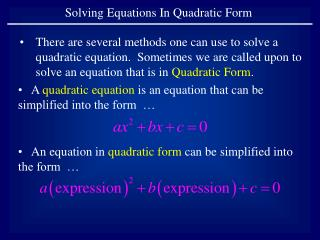# Solving Equations In Quadratic Form - PowerPoint PPT PresentationDownload PresentationDownload Presentation## Solving Equations In Quadratic Form

- - - - - - - - - - - - - - - - - - - - - - - - - - - E N D - - - - - - - - - - - - - - - - - - - - - - - - - - -
##### Presentation Transcript

1. Solving Equations In Quadratic Form • There are several methods one can use to solve a quadratic equation. Sometimes we are called upon to solve an equation that is in Quadratic Form. • A quadratic equation is an equation that can be simplified into the form … • An equation in quadratic form can be simplified into the form …

2. Example 1: Solve the equation Move all terms to the left side. This equation is now in quadratic form.

3. We will solve the equation with what is called a u-substitution. Let u = expression. The equation now takes the form of a quadratic equation by substituting u for the expression.

5. Since the original equation was in variable x, our solutions need to be in variable x. Taking the solutions of u and combining them with the original u-substitution equation we get …

6. Solving these two quadratic equations … … we now have the solution to the original equation.

7. Example 2: Solve the equation Note that this equation is in quadratic form.

8. Use a u-substitution. Let u = expression. The equation now takes the form of a quadratic equation by substituting u for the expression.